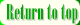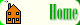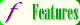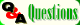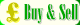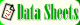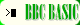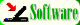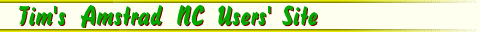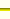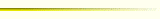How BBC BASIC Stores Your Programs

By Tim Surtell

When you type a program into the BBC BASIC interpreter on your Notepad or Notebook, the lines are not stored exactly as you have typed them. When you press ENTER after typing a line, the interpreter reads the line and searches for any BASIC commands. For each command it finds, it replaces the word with a single byte 'token' for that command. Each command has a unique token so that BASIC does not get confused.

A list of the tokens can be found on the BASIC Commands Index page.

Replacing each command with a token has two advantages for BBC BASIC:

• The tokenised program takes up less memory in the computer since a command that might be 5 characters long such as 'PRINT' becomes a single byte (241). The program will run faster since BASIC only has to analyse one byte of information for each command rather than finding out if there is a string of characters making up a command (ie., the hard work is done when the line is typed in rather than when the program is run.)
• The BBC BASIC system goes further than just tokenising the commands. Line numbers are also changed into two bytes -- the first gives the number of 256's that can be divided into the line number. The second gives the remainder of this calculation. Therefore, if the Most Significant Byte (MSB) is 2 and the Least Significant Byte (LSB) is 88 then the line number is (2*256)+88 = 600.
The line numbers after GOTO and GOSUB are also coded in this way.
Other parts of program lines such as numbers, variable names, and other symbols are left as they are.

Also, the first byte in each line tells the interpreter how many bytes there are in the line. This means that the maximum number of characters in a line is 255. The end of a line is always marked with the code 13, which is the CARRIAGE RETURN character. The end of the program is marked by having the total number of bytes in the line equal to 0 and the two following bytes equal to 255. The diagram below illustrates all of this: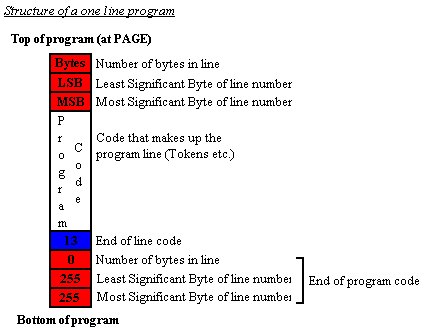As an example, consider the following two line program :

10 PRINT"Ten second timer"
20 TIME=0:REPEAT:UNTIL TIME>1000:VDU 7

When typed in, this is stored as follows: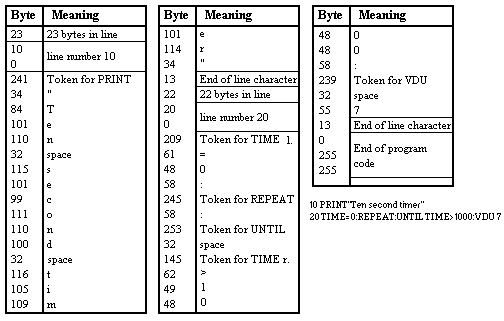Note that there are two tokens for the command TIME : one for when it is left of an operator (TIME=0) and one for when it is right of an operator (UNTIL TIME>1000). Note also that there are two unneccasary spaces in the program. These, and other which you would not be able to remove when typing in a program, can be removed using the compacter program below.

BBC BASIC compactor

This program can be run after you have finished writing a program to compact it so that is uses less memory. The program does several things to save memory :

• It removes any spaces that are not needed.
• It changes all variable, procedure and function names to 1 or 2 characters.
• It removes REM lines and variables after NEXT.
• COMPACT.TXT ... 4kb

Running the program

The program uses the PAGE variable which specifies the memory address where the first byte of your program will be found.

Because you will need to change PAGE, BACKUP ANY FILES ON YOUR MACHINE BEFORE RUNNING THE PROGRAM.

• Press FUNCTION-B to enter BBC BASIC.
• Load in the program you want to compact.
• Type PRINT PAGE to find the current value of PAGE. Note it down.
• Type PAGE=&5000 to set page to 5000h.
• Load in the COMPACT program. The program will be loaded from the memory address 5000h, so the original program will still be available at the old value of PAGE.
• Run the program and enter the value of PAGE that you noted earlier. The program will run and show it's progress by displaying the line number of the program line it is working on.
• When the program is finished, type PAGE=**** where **** is the value of PAGE that you noted down.
• List the program and you should find it compacted. Save the program.
• Differences between the NC and the BBC Micro

Although the NC uses the same tokens for commands as the BBC Micro does, programs written on the BBC Micro will not load on the NC because the order of the line number and number of bytes is different, as are the codes at the end of a program . Figure 3 shows the differences: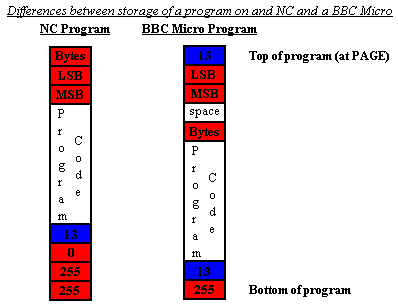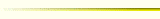Compacter program taken from Advanced programming techniques for the BBC Micro by Jim McGregor & Alan Watt, page 342. ISBN : 0-201-14059-4
BBC BASIC token list taken from the BEEBUG reference card© 1997 – 2023 Tim Surtell Tim's Amstrad NC Users' Site www.ncus.org.uk